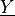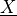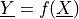# Composite random vector¶

In this example we are going to create a random variablewhich realizations are the images of the realizations of another random vectorby a function.```import openturns as ot

ot.Log.Show(ot.Log.NONE)
```

Create a random vector based on a distribution

```dist2d = ot.Normal(2)
X = ot.RandomVector(dist2d)
```

Create the function

```f = ot.SymbolicFunction(["x1", "x2"], ["x1 + x2", "x1*x2"])
f
```

[x1,x2]->[x1 + x2,x1*x2]

Create the composite random vector

```Y = ot.CompositeRandomVector(f, X)
```
```Y.getSample(5)
```
y0 y1 -2.346634 1.367485 -1.279833 0.3801989 1.09672 -0.04583323 2.298412 1.153096 0.7112375 0.06075101